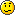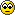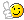# I'm stuck...

Member Posts: 16 ■□□□□□□□□□
Question:
What is the maximum number of hosts in a /19 subnet?

8190 is the total number of hosts (2^14 - 2)

I would have thought since /19 gives you:

11111111111111111110000000000000

...which gives me 13 unmasked bits or zeros. So I thought from the books I am reading that the formula would give me (2^13 - 2). What am I missing?:

Thanks,

Joe

• Member Member Posts: 39 ■■■□□□□□□□
2^1=2
2^2=4
2^3=8
2^4=16
2^5=32
2^6=64
2^7=128
2^8=256
2^9=512
2^10=1024
2^11=2048
2^12=4096
2^13=8192

should be (2^13-2) Hope that helps.
• Member Posts: 16 ■□□□□□□□□□
gramacorp wrote: »
2^1=2
2^2=4
2^3=8
2^4=16
2^5=32
2^6=64
2^7=128
2^8=256
2^9=512
2^10=1024
2^11=2048
2^12=4096
2^13=8192

Ah, I think you are right. It does make it tough when the book has a slip up!It is problem number 7, end of the chapter review on subnetting. Mike Meyers, Network+, All-In-One, Fourth Edition. I guess my confidence is still lacking, because I didn't take it far enough to test what I thought to be right.Thanks for your help!